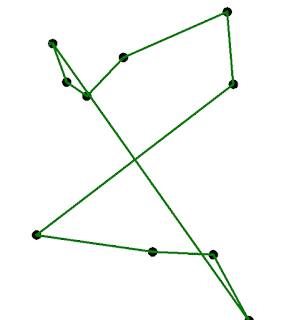# Brute force Travelling Salesman Problem in Python

I did not make it into work today. A blanket of snow - dubbed the Pest from the West - hit the North of England causing gridlock in many areas. Instead, I got to stay at home and write a Travelling Salesman simulation in Python.

The Travelling Salesman Problem (TSP) is a problem in Computer Science used to demonstrate intractability and optimisation. In short, imagine a salesman who wishes to visit every one of N cities exactly once each. The salesman does not care which order he visits the cities as he is equally proficient in applying is selling skills in them all regardless. All that matters is that he takes the shortest route between them all before returning back to the start, where, presumably Alan Sugar is waiting in the boardroom to fire him if he has failed in this task.

The Python 3 code below will run a TSP simulation by attempting to solve the problem by brute force. This is, of course, the worst method for solving the problem, being factorial of N time complexity, but it is presented here in the hope that it is of some use to students.A solution for 10 citiesThe simulation in mid-animationA solution for 9 citiesHere is a photo of me failing to complete the TSP for 2 cities.
The Python code can be copied from below, or you can download it from my OneDrive. Enjoy!

``````
# Travelling Salesman by Brute force
# version 2.1
# 2018-03-08

import itertools
import turtle
import time
import math
import random

class Salesman(object):
"""Travelling Salesman by Brute force"""
# in this program a route is represented by a list of coordinates
# where each coordinate is a tuple containing an x,y coordinate
# in the range 0-1000,0-1000
def __init__(self):
self.__city = [] #list for holding city coords
self.__num = 0 # number of cities in simulation
self.__animate = False # True if should show animations
self.__speed = 100 # Speed of animations 100 is fastest
self.__wn = turtle.Screen() # turtle window
turtle.setworldcoordinates(0, 0, 1000, 1000)
self.__wn.title("TRAVELLING SALESMAN")
self.__cityPen = turtle.Turtle() # pen for drawing cities
self.__cityPen.shape("circle")
self.__cityPen.hideturtle()
self.__routePen = turtle.Turtle() # pen for drawing routes
self.__routePen.pensize(5)
self.__route = [] # list of possible routes
self.__bestRoute = None # current best route
self.__bestLength = 1000000 # a big number

def main(self):
"""Main test program"""
self.__title()
self.__setup()
self.__generate()
self.__showCities()
try:
self.__getRoutes()
t0 = time.clock() # start tinmer
for i in self.__route:
thisLength = self.__calculateLength(i)
if thisLength <= self.__bestLength:
self.__bestLength = thisLength
self.__bestRoute = i # store the best route so far
if self.__animate:
self.__showRoute(i)
time.sleep(0.01)
self.__routePen.clear()
#show the best route
print("\nDone. Showing best route")
print(time.clock() - t0, "seconds")
self.__showRoute(self.__bestRoute, "green")
input()
self.__cityPen.clear()
self.__routePen.clear()
self.__routePen.hideturtle()
self.__cityPen.hideturtle()

except Exception as e:
print("Ooops, that was an error. Too many cities?")

def __setup(self):
"""Gets users choices for the simulation"""
ok = False
while not(ok):
try:
num = int(input("Enter number of cities: "))
if num>1 and num < 11:
self.__num = num
ok = True
else:
print("Invalid!")
except Exception as e:
pass
choice = input("Show animation for all? (Y/N): ")
if choice.lower() in "yes":
self.__animate = True
ok = False
while not(ok):
try:
speed = int(input("Select speed (1-10): "))
if speed >= 0 and speed <= 10:
self.__speed = speed ** 2
ok = True
else:
print("Try again")
except Exception as e:
pass

def __generate(self):
"""Creates random cities"""
for i in range(self.__num):
x = random.randint(0,1000)
y = random.randint(0,1000)
self.__city.append((x,y))

def __showCities(self):
"""Display cities on the canvas"""
self.__cityPen.speed(0)
self.__cityPen.penup()
for i in range(self.__num):
self.__cityPen.goto(self.__city[i],self.__city[i])
self.__cityPen.stamp()

def __getRoutes(self):
"""Find all combinations of routes"""
trylist = list(itertools.permutations(self.__city))
#remove duplicates
for i in trylist:
if i == trylist:
self.__route.append(i)

def __showRoute(self, this, color="red"):
"""Show a route passed as paramter on the canvas"""
self.__routePen.color(color)
self.__routePen.speed(self.__speed)
self.__routePen.penup()
for i in this:
self.__routePen.goto(i,i)
self.__routePen.pendown()
self.__routePen.goto(this,this)

def __calculateLength(self, this):
"""Returns the length of route 'this'"""
d = 0
for i in range(1,len(this)):
d += math.sqrt( (this[i]-this[i-1])**2 + (this[i]-this[i-1])**2)
return d

def __title(self):
"""Displays the title"""
print("Travelling Salesman Problem")
print("      (by brute force)")

while True:
app = Salesman()
app.main()

``````